# Tri-Lasers for Felines

The aim is to entertain cat(s) with a moving laser game. it activates when the cat is around, and in case of attack, it shut down!

BeginnerFull instructions provided4 hours3,097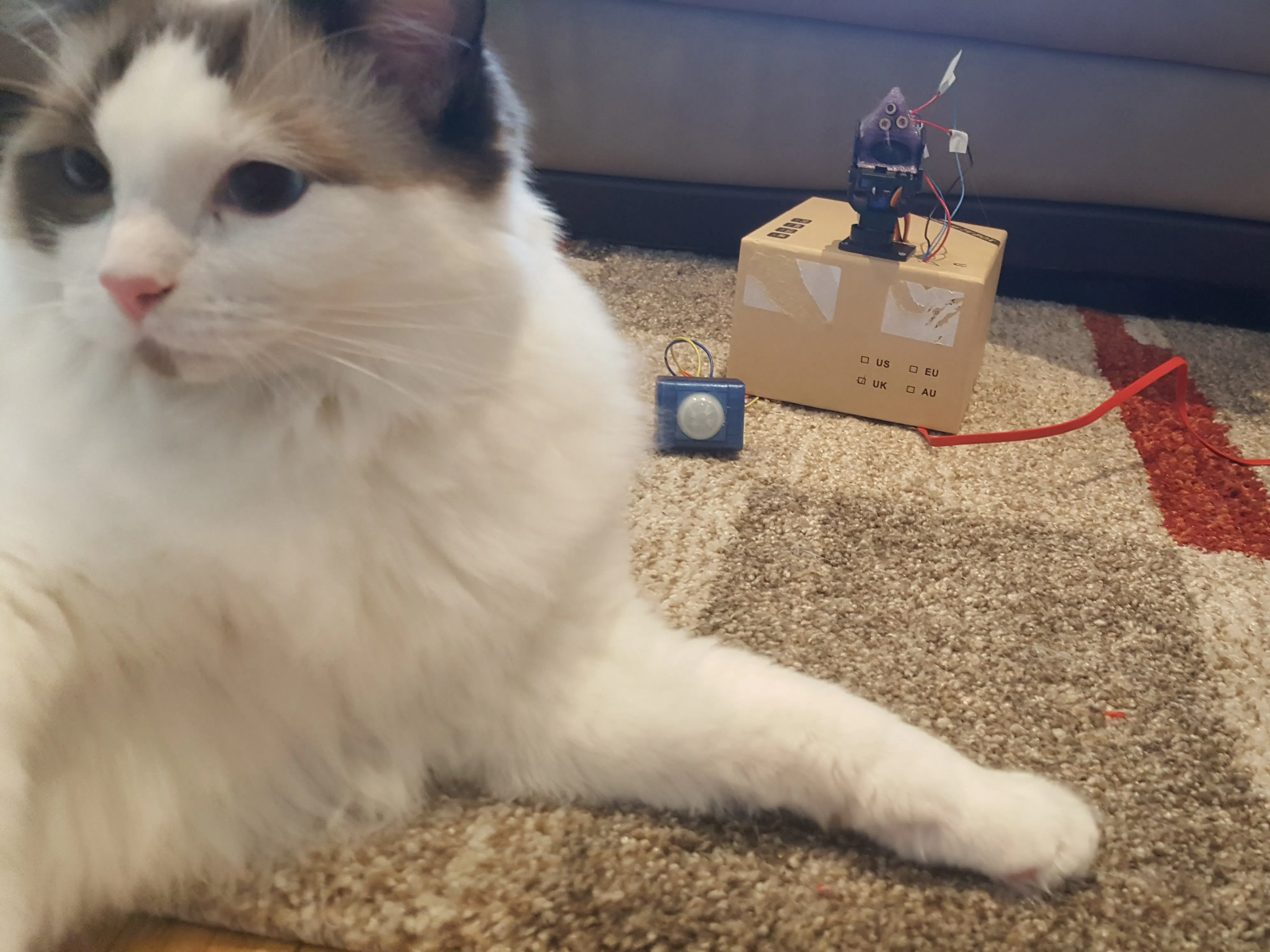## Things used in this project

### Hardware components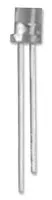Laser Diode, 2 Pins
×3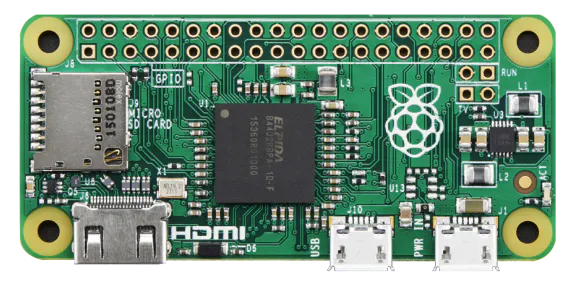Raspberry Pi Zero
×1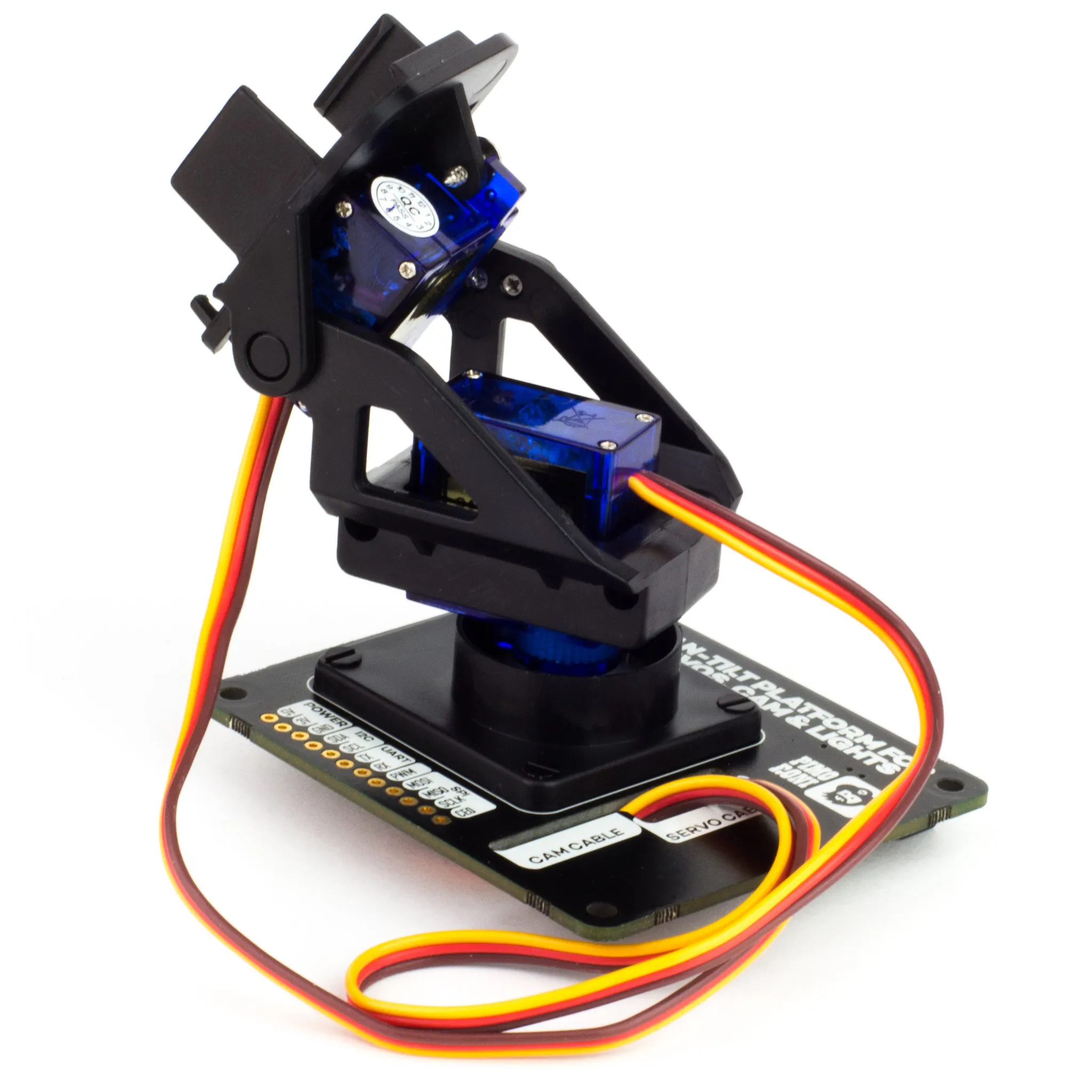Pimoroni Pan-Tilt HAT
×1Tilt Switch, Encapsulated
×1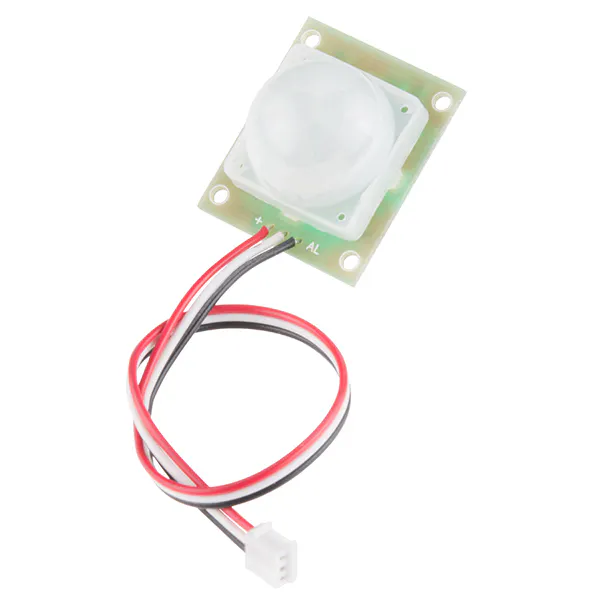PIR Motion Sensor (generic)
×1Jumper wires (generic)
×10
 generic cardboard boxes
×1

### Hand tools and fabrication machines3D Printer (generic) optionalSoldering iron (generic) optionalHot glue gun (generic) optional

## Schematics

### circuit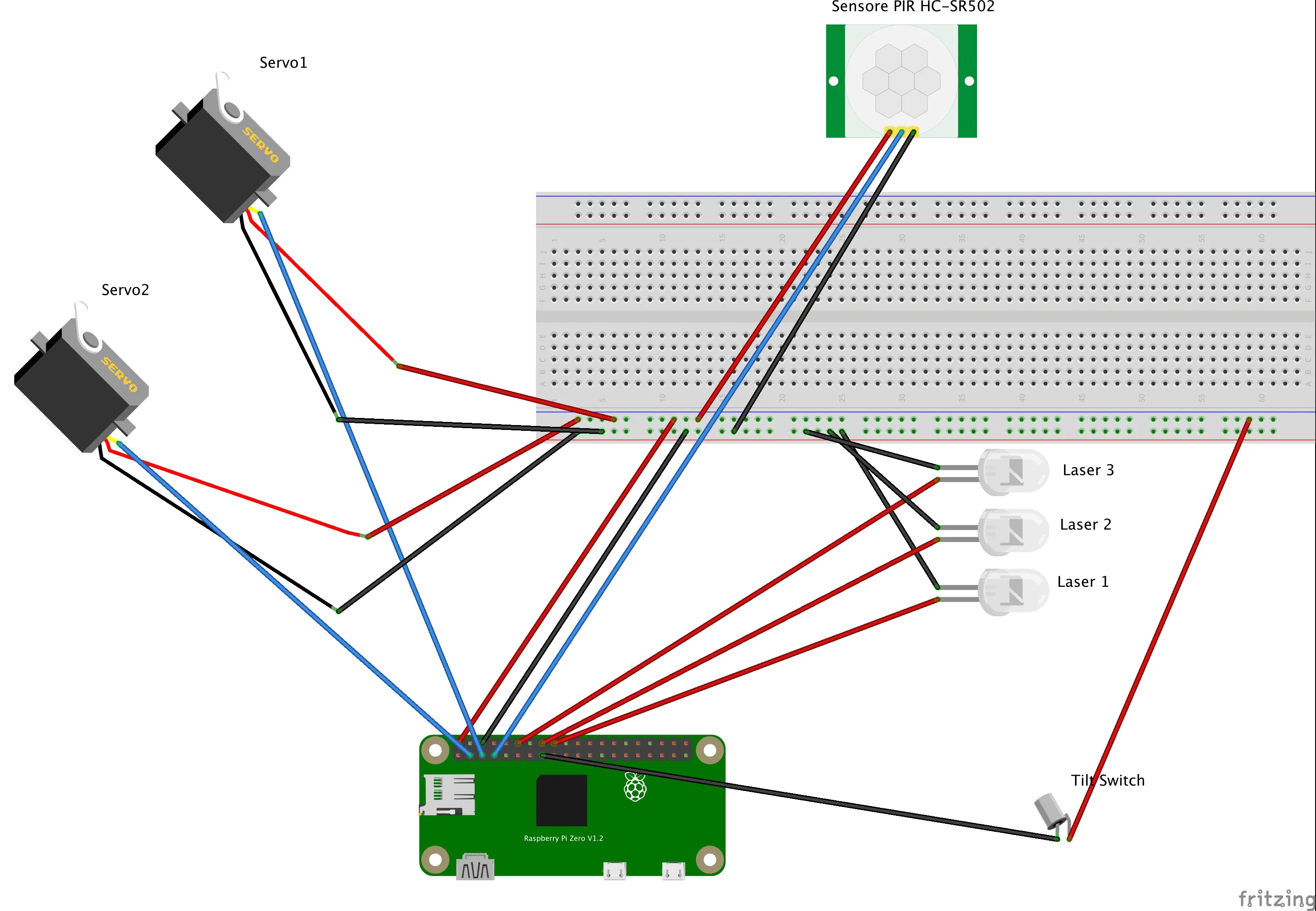## Code

### main code

Python
Please check the github repository for an update version of the code.
```#!/usr/bin/env python

import logging
import random
import time

import pantilthat
from gpiozero import LED
from gpiozero import MotionSensor
from gpiozero import Button

DELAY = 1
TIME_ACTIVITY = 60  # is in seconds
HORIZONTAL_RANGE = 90
VERTICAL_RANGE = 90
SWITHCH_ON_LED = 0
LOG_FILENAME = 'logs/CatFitBot.log'

# Create a custom logger
logger = logging.getLogger(__name__)
handler2 = logging.StreamHandler()  # handler for routine view
handler1 = logging.FileHandler(LOG_FILENAME)

# Create handlers
format1 = logging.Formatter('%(asctime)s - %(name)s - %(message)s')
format2 = logging.Formatter('%(name)s - %(message)s')
handler1.setFormatter(format1)
handler2.setFormatter(format2)

# Create formatters and add it to handlers

logger.log(level=40, msg="The Application is starting")

# Hardware settings
pir = MotionSensor(4)
button = Button(22, pull_up=False)  # the tilt_switch sensor
led3 = LED(18)
led1 = LED(24)
led2 = LED(23)

def cat_attack():
"""
check the status of the tilt switch, in case it is true,
the application assume a cat attack and exit. to stop any moving part and led activity.
hoping to be spare by the cat.
:return: none
"""
if button.is_pressed:
logger.log(level=40, msg="No Cat attack has been logged")
print("No Cat attack has been logged")
else:
print("exit()")

def led_random(number=1):
"""
:param number: maximum number of led(s) simultaneously switch on.
in case of zero only one ld is used.
:return: none
"""
if number == 0:
uplimit = 1
elif number == 1:
uplimit = 3
elif number == 2:
uplimit = 6
else:
uplimit = 7

k_ = random.randint(1, uplimit)
time.sleep(DELAY * k_ / 20.)
if k_ == 1:
led1.off()
led2.off()
led3.on()
elif k_ == 2:
led1.off()
led2.on()
led3.off()
elif k_ == 3:
led1.on()
led2.off()
led3.off()
elif k_ == 4:
led1.on()
led2.on()
led3.off()
elif k_ == 5:
led1.on()
led2.off()
led3.on()
elif k_ == 6:
led1.off()
led2.on()
led3.on()
elif k_ == 7:
led1.on()
led2.on()
led3.on()

while True:
led1.off()
led2.off()
led3.off()
print("Waiting")
logger.log(level=40, msg="Waiting to start")
pir.wait_for_motion()
logger.log(level=40, msg="Started the post wait")

led_random(number=SWITHCH_ON_LED)
print("Led 1 on")

# Reset the time
t = time.time()
t2 = time.time()

a1 = random.randint(40, 90) * (-1)

#  k = random.randint(1, 10)
b1 = random.randint(0, 80) - 40
while t2 - t < TIME_ACTIVITY:
logger.info('routi for 1 minute')
print("Time: t2--> {} t--> {} delta--> {}".format(t2, t, t2 - t))
# Get th
a2 = random.randint(40, 90) * (-1)
b2 = random.randint(0, 80) - 40
print("{}  {}".format(a1, b1))
print("{}  {}".format(a2, b2))

a_step = (a1 - a2) / 10
b_step = (b1 - b2) / 10

# micro movement
for i in range(10):
cat_attack()  # check for the cat activity....
print("init {} finale{} step {} posizione {} delta {}".format(a1, a2, i, int(a1 - i * a_step), a_step))
pantilthat.pan(int(b1 - i * b_step))
pantilthat.tilt(int(a1 - i * a_step))

# Two decimal places is quite enough!
print(round(b1, 2))

led_random(number=SWITHCH_ON_LED)
time.sleep(DELAY * 0.1)
a1 = a2
b1 = b2
k = random.randint(1, 10)

time.sleep(DELAY * 5 / k)
t2 = time.time()
```

## Credits

### enzo

8 projects • 9 followers